# McGraw Hill Math Grade 3 Chapter 8 Lesson 9 Answer Key Comparing Fractions with the Same Denominator

## McGraw-Hill Math Grade 3 Answer Key Chapter 8 Lesson 9 Comparing Fractions with the Same Denominator

Compare

Compare the fractions. Write >, <, or =.

Question 1.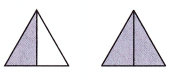$$\frac{1}{2}$$1
$$\frac{1}{2}$$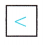1
According to the pictures shown above 1/2 is less than 1.

Question 2.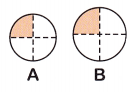$$\frac{1}{4}$$ of A$$\frac{1}{4}$$ of B
The shape of both the circles is the same but the size is different.
Circle A is less than Circle B.
$$\frac{1}{4}$$ of A < $$\frac{1}{4}$$ of B

Question 3.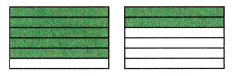$$\frac{5}{6}$$ of A$$\frac{2}{6}$$ of B
$$\frac{5}{6}$$ of A > $$\frac{2}{6}$$ of B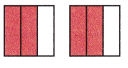$$\frac{2}{3}$$ of A$$\frac{2}{3}$$ of B
$$\frac{2}{3}$$ of A = $$\frac{2}{3}$$ of B